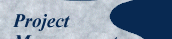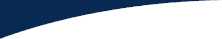Published here December 2004.

## Brenda Taylor Tries to Summarize

Hi all

Thank you all for your feedback in this regard. I have included the various responses received. As you can see, there is consensus amongst some of you regarding the use of the budget at completion x % complete (even though this is not consistent with current PMI thinking) and planned value x % complete (which is in line with current PMI texts). However, I would not necessarily agree with the use of either of these in extrapolating to ascertain the actual cost and schedule performance indicators for a project and the estimate at completion for cost and time considering current project progress.

Surely it stands to reason that if I am spending more of the budget than I had anticipated spending, to get the same amount of work done, my project is going to be over budget or I am going to have to pull in the belt of my project and so cost more time? Thus, the project is not earning the value it is meant to be earning.

Or the corollary of this would be if I am behind schedule but have spent the amount of money I had planned to spend to this date, the value I am deriving for my project is lower because I am getting less work for the same amount of money.

Quentin, when you say that the earned value represents the completed authorized tasks, and the same budget for the completed tasks, why use the planned value to calculate this? Why dont you use the entire budget for the project (BAC) and multiply it by the actual percentage complete of the total authorized tasks. This would calculate how much the completed authorized tasks should have cost, but would not necessarily tell you how much value is being added to the project when compared to project spend. Using the planned value would not give you the correct EV because that would indicate that you had planned to spend a certain amount and you only have a certain percentage of that amount of work to show for that planned spend. What if you are actually on schedule project-wise and budget-wise?

Besides this argument, the simple mathematics of it does not calculate accurately when using the EV = PV x % Com; SV=EV-AC especially when using a project that is on schedule and on budget.

I repeat my example:

EV = PV x % Com
SV = EV  PV
Therefore: SV = (PV x % Com.) - PV [derived by substituting PV x % Com for EV]

If a project has a budget of R10,000 and to date we had planned to spend R7,000 and we have spent R7,000 and the project is planned to be 70% complete and is 70% complete then the schedule variance and the cost variance should be 0 because, although it is only 70% complete, it is, in fact, on schedule and there is, therefore no variance to the schedule evident.

According to this formula, however, this is not true. If I were to input the values - as indicated by the formula - the schedule variance would reflect as follows:

EV=7,000 x 70%
= R4,900
SV = R4,900 - R7,000
= R2,100 which is not true because this project is on budget and on schedule. There is no schedule or cost variance.

Okay, so let's work out the SPI, because we know that if the project is running according to schedule and budget, then the SPI should be 1 or 100%, right?

SPI = EV/PV
=4900/7000

Which does not equal 1 or 100%.

If however, we use BAC to calculate the earned value as Frank and Louise suggest, this tells us how much of the work we have done and should have cost us but not necessarily the actual value our project is earning as a result of spending the money were spending.

Im not saying that either of these are correct or incorrect, Im saying that we need to consider what it is were trying to calculate or end up with by doing any of these calculations. When I report to my management that my project has an earned value of R4,900 and Ive actually spent R7,000 theyre going to ask many questions that I will not be able to answer except by directing them to the gospel according to PMI. When they ask me why I am only 70% complete and Ive already spent 95% of the budget (which may be something I have had to do because of expensive equipment or more expensive resources earlier on in the planned project) and I tell them Ive actually only earned a value of R7,000 for their R9,500 spent, theyre going to ask me questions which I will only be able to answer by directing them to earlier versions of the gospel according to PMI.

Your comments and further input would be most welcome. Thank you very much for all your assistance with this.

Kindest regards
Brenda